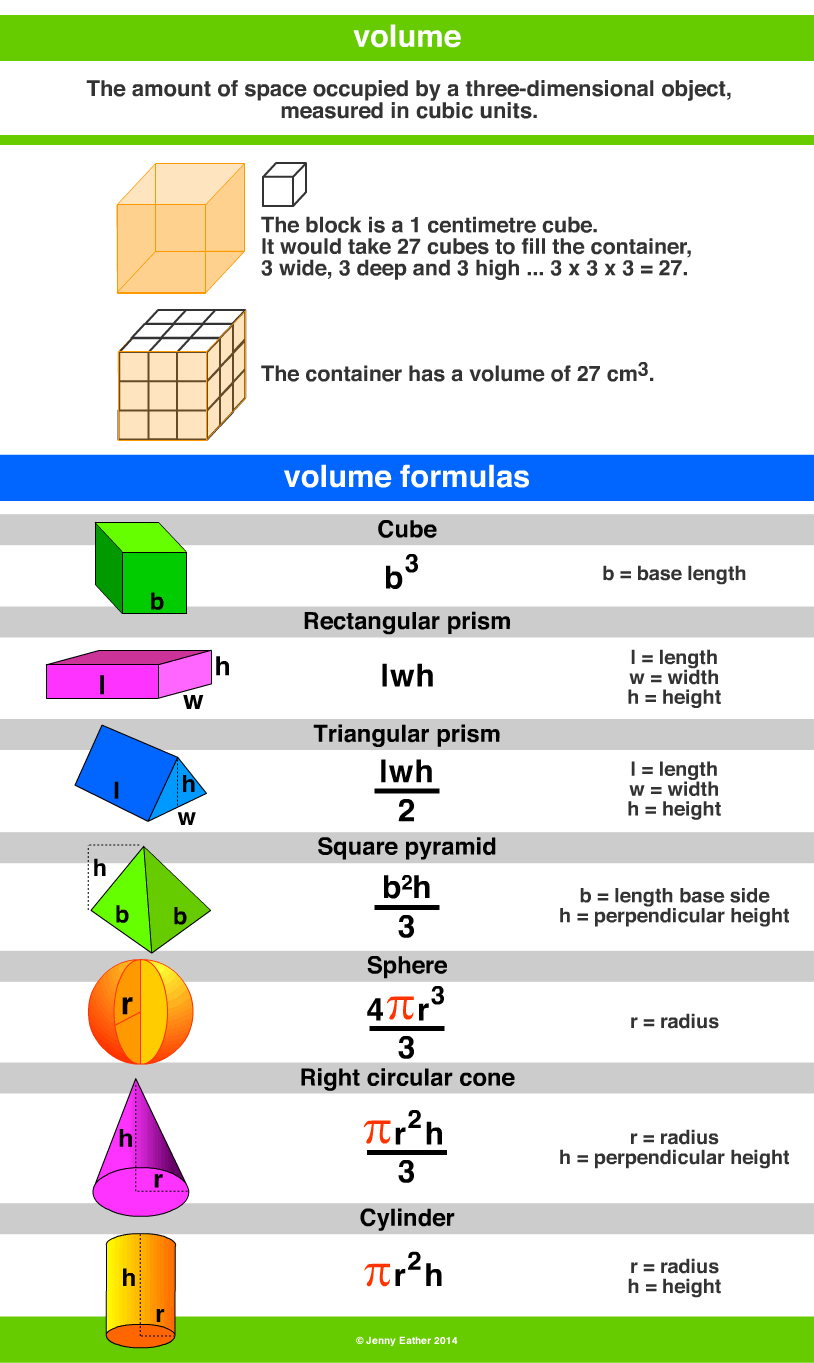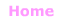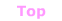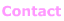Aa Bb Cc Dd Ee Ff Gg Hh Ii Jj Kk Ll Mm Nn Oo Pp Qq Rr Ss Tt Uu Vv Ww Xx Yy Zz

Vv

volume

• amount of space occupied by an object.

EXAMPLES:Metric Units ~ Volume

1000 cubic millimetres = 1 cubic centimetre
1000 cubic centimetres = 1 cubic decimetre
1000 cubic decimetres = 1 cubic metre
1 000 000 cubic centimetres = 1 cubic metre

Abbreviations
cubic millimetre mm3 cubic centimetre cm3
cubic metre m3

Imperial/Customary Units ~ Volume

1728 cubic inches = 1 cubic foot
27 cubic feet = 1 cubic yard

Imperial/Customary ~ Fluid Volume

8 fluid ounces = 1 cup (US)
10 fluid ounces = 1 cup (UK)
16 fluid ounces = 1 pint (US)
20 fluid ounces = 1 pint (UK)
2 pints = 1 quart
4 quarts = 1 gallon

1 gallon = 4 quarts, 8 pints, 16 cups© Jenny Eather 2014. All rights reserved.